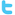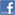Genesis Insoft The name you can TRUSTHome  |  Contact Us |  Careers
Java Question Bank / FAQs

This is a mock Exam based on the Objectives for the Sun Java Programmers Exam. It is created by Genesis InSoft Limited (admin@genesisinsoft.com) and may be freely distributed so long as it is unmodified. Please email us if you have any corrections or comments.

##### Question 1

The Java disassembler option, which is used to disassemble the source code and display the byte code produced by the compiler is

1. javap -dc
2. javap -a
3. javap -c
4. javap -l
##### Question 2

What is the output of the program?

outer:for (int i = 0; i < 2; i++) {
for (int j = 0; j < 3; j++) {
System.out.println ("i = " + i + " j = " + j);
if (i == j) {
continue outer;
}
}
}

1. I=0 j=0 , I=0 j=0 and I=0 j=2
2. I=0 j=0 , I=0 j=1 and I=1 j=2
3. I=0 j=0 , I=1 j=0 and I=1 j=1
4. I=0 j=1 , I=0 j=2 and I=2 j=0
##### Question 3

Consider the following code:

1.    public class Test3 {
2.        static int total = 10;
3.        public static void main (String args []) {
4.             new Test3();
5.        }
6.        public Test3() {
7.            System.out.println("In test");
8.            System.out.println(this);
9.            int temp = this.total;
10.          if (temp > 5) {
11.              System.out.println(temp);
12.          }
13.      }
14.  }

What is the output of the above program?

1. The compiler reports and error at line 2.
2. The compiler reports an error at line 9.
3. The value 10 is one of the elements printed to the standard output.
4. The class compiles but generates a runtime error
##### Question 4

What is the output of the program?

public class Xor {
public static void main(String args[]) {
byte b = 10;
byte c = 15;
b = (byte)(b ^ c);
System.out.println (b);
}
}

1. 25
2. 5
3. 10
4. 15
##### Question 5

True or False: Readers have methods that can read and return floats and doubles.

1. True
2. False
##### Question 6

The "System.out" object used for the standard input oprations is instantiated from which class?

1. System class
2. OutputStream class
3. PrintStream class.
4. Object class
##### Question 7

Does the code fragment below compile successfully? If so, is line 2 executed?

if ("Hedgehog".startsWith("Hedge"))
System.out.println("Line 2");

1. code does not compile
2. code compiles, line 2 does not executed
3. code compiles, line 2 executed
4. none
##### Question 8

public class Base {
public int method(int i) {
System.out.println("Value is " + i);
return 0;
}
}

public class Sub extends Base {
public int method(int j) {
return (method("method("+j+")"));
}
public int method(String s) {
System.out.println("I was passed " + s);
return s.length();
}
public static void main(String args[]) {
Base b1 = new Base();
Base b2 = new Sub();
b2.method(b1.method(b2.method(5)));
}
}

What is the output when the main method of the class Sub is run?

1. I was passed method(5), value is (9), I was passed method(0)
2. I was passed method(5), value is (9), I was passed method(5)
3. I was passed method(0), value is (9), I was passed method(5)
4. I was passed method(5), value is (0), I was passed method(5)
##### Question 9

One Java application can have more than one main () method.
State which following statement is correct?

1. We can not put more than one main method
2. We can put more than one main method, but all methods should have same signature
3. We can put more than one main method, but all methods should have different signature
4. We can put more than one main method and signatures can same or different
##### Question 10

What is the output when the main method of the class test is run?

public class test{
public static void main(String arg[]) {
int a = 5;
a+=++a;
System.out.print (a);
}
}

1. 12
2. 11
3. 10
4. 13
##### Question 11

Consider the following code:

public class prg {
static int A;
public static void main(String a[]) {
boolean x;
if((x=(++A==0)? false:true))
if((x=(++A==0)? true: false))
System.out.println (++A);
else
System.out.println(A + 10);
else
System.out.println(A + 20);
}
}

What is the output when the main method of the class test is run?

1. 2
2. 20
3. 11
4. 12
##### Question 12

Consider the following code:

public class prg {
public static void main(String a[]) {
switch ('\0') {
case '0': System.out.print(" 0 ");
case '1': System.out.print(" 1 ");
}
}
}

What is the output when the main method of the class test is run?

1. 0
2. 0 1
3. no output
4. compilation error
##### Question 13

Consider the following code

public class prg {
static int cal(int x, int y) {
return(x + 2 * y);
}
public static void main(String a[]) {
int x = 0, y = 2;
System.out.println (cal (x += 5, y = x = 9));
}
}

What is the output when the main method is run?

1. 32
2. 19
3. 23
4. 18
##### Question 14

Comment on the  following declarations?
String str;
String str=" " ;

1. both are same and both refer to null.
2. they are different with the first declaration as a null String Object and the second one as null String.
3. they are different with the difference that the second one is instantiated and initialised to null and the first one is instantiated and refers to some garbage value.
4. None of the above.
##### Question 15

Consider the following code:

public class Xor {
public static void main(String args[]) {
final int x=4;
if ( x++ != 0 )
System.out.print ("x val "+x);
}
}

What is the output when the main method of the class test is run?

1. can be compiled , no output
2. can be compiled , output is 5
3. compilation error
4. no output
##### Question 16

Consider the following code:

class xyz {
public String myname;
}

public class test {
public static void main(String args[]) {
xyz a = new xyz();
xyz b = a;
b.myname = "newname";
System.out.print(" str1 "+a.myname+" str2 "+b.myname);
}
}

What is the output when we run the above program?

1. newname, newname
2. no value, newname
3. no output
4. newname, no value
##### Question 17

Which one statement is true about the code fragment below?

import java.lang.Math;
public class math {
public static void main(String arg[] ){
Math myMath = new Math();
System.out.println("cosine of 0.123 = " + myMath.cos(0.123));
}
}

1. compilation error line no 4
2. compilation error line no 5
3. no compilation error, during the execution, an exception is thrown at line 5
4. no compilation error and no exception is thrown and display the output

Question 18

What does the access Specifier " private protected " mean ?

1. The method is accessible by the class only.
2. The method is accessible by the class and its subclasses but the instances of the class or its
subclass cannot.
3. The method is accessible by the class and classes which are available in the same package.
4. There is no such access specifier
##### Question 19

What is the ouput when the following code is executed?

public class Short {
public static void main(String args[]) {
StringBuffer s = new StringBuffer("Hello");
if((s.length() > 5) && (s.append(" there").equals("False"))) ;
System.out.println("value is " + s);
}
}

1. The output: value is Hello
2. The output: value is Hello there
3. compile error at line no 4
4. Null pointer exception
##### Question 20

Which of the following determines if the specified character is a "Java" letter or digit, that is, the character is permissible as a non-initial character in an identifier in the Java language.

1. isLetterorDigit( char )
2. isletterOrdigit( char );
3. isJavaLetterOrDigit( char )
4. isJavaletterOrDigit( char )